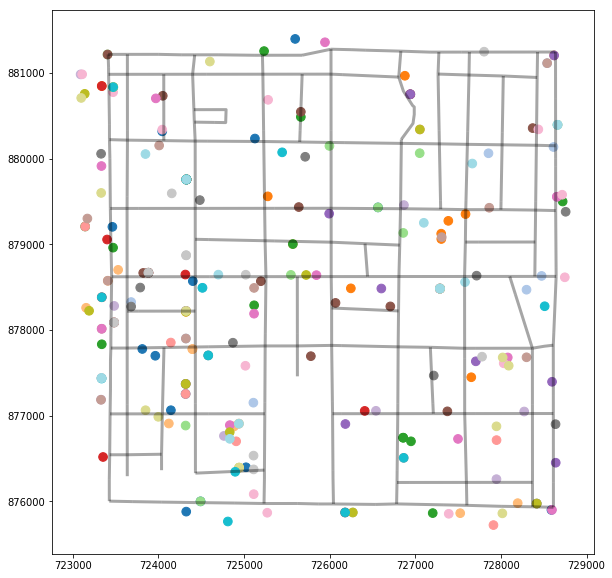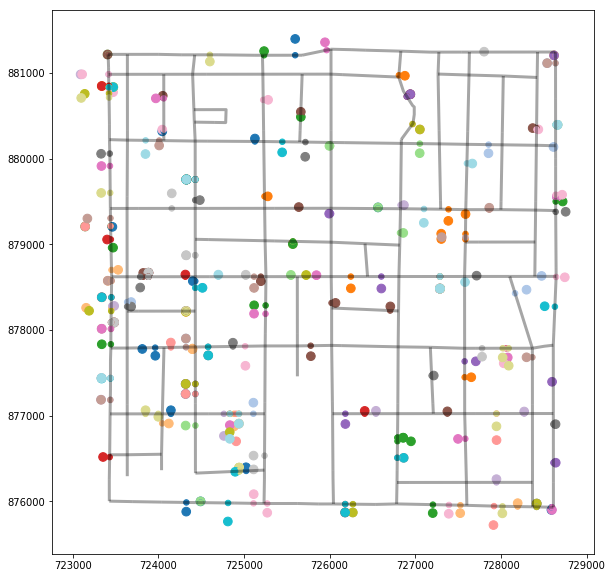# Snapping point to segments¶

In :
import os
last_modified = None
if os.name == "posix":
last_modified = !stat -f\
"# This notebook was last updated: %Sm"\
Snapping_Demonstration.ipynb
elif os.name == "nt":
last_modified = !for %a in (Snapping_Demonstration.ipynb)\
do echo # This notebook was last updated: %~ta

if last_modified:
get_ipython().set_next_input(last_modified[-1])

In [ ]:
# This notebook was last updated: May 13 20:04:46 2019


In :
import numpy as np
from libpysal import examples
import geopandas as gpd
import spaghetti as spgh
from shapely.geometry import Point

%matplotlib inline

__author__ = 'James Gaboardi <[email protected]>'


## Segments¶

In :
streets = examples.get_path("streets.shp")
streets = gpd.read_file(streets)


## Points¶

In :
crimes = examples.get_path("crimes.shp")
crimes = gpd.read_file(crimes)
np.random.seed(1)
crimes['geometry'] = np.random.permutation(crimes['geometry'])


## Initial plot¶

In :
base = streets.plot(figsize=(10,10), color='k', alpha=.35, linewidth=3)
crimes.plot(ax=base, cmap='tab20', markersize=75)

Out:
<matplotlib.axes._subplots.AxesSubplot at 0x1160544e0># Network¶

In :
net = spgh.Network(in_data=streets)


## Snap point onto nearest segments¶

In :
net.snapobservations(crimes, 'crimes')


## Create geopandas.GeoDataFrame objects of snapped points¶

In :
snapped_gdf = spgh.element_as_gdf(net, pp_name='crimes', snapped=True)


## Original point coordinates, snapped point coordinates¶

In :
original = net.pointpatterns['crimes'].points
print(original['coordinates'], snapped_gdf.geometry.coords[:])

(726942.0000000028, 880750.9999999988) [(726901.5389320733, 880725.212354287)]


## Snapped points plot¶

In :
base = streets.plot(figsize=(10,10), color='k', alpha=.35, linewidth=3)
crimes.plot(ax=base, cmap='tab20', markersize=75)
snapped_gdf.plot(ax=base, cmap='tab20', markersize=30)

Out:
<matplotlib.axes._subplots.AxesSubplot at 0x118701048>